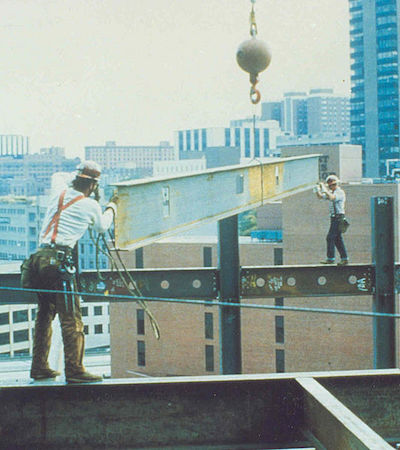# Gravity SoundA construction worker's helmet slips and falls when he is $78.4$ meters above the ground.

He hears the sound of the helmet hitting the ground $4.23$ seconds after it slipped. Find the speed of sound in air.

Details and assumptions:
1) Take the acceleration due to gravity as $g = 9.8\frac{m}{s^2}.$